Next: Trial Orbits Up: Implementation of the AVP Previous: Implementation of the AVP

## The AVP Code

A description of the code used to locate the minimum points of the action will be given in this subsection, including some of its specifications. The code, inventively called AVP, was written in the Fortran 90/95 programming language, consisting of subroutines, functions, and modules. The complete code will be given in Appendix A, where also a more detailed description is given of the structure of the code dependent on the programming language.

Before looking into the details of the AVP code, it should be noted that all parameters and variables are scaled according to mass, distance, and time given in the following units``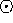'' indicating solar value. The reason for this scaling is to make the values used in the calculation as close to unity as possible, hence reducing the risk of roundoff errors. The resulting range of the variables, in this case the coefficients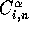in equation (3.21), is about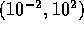. For some of the optimizing methods this scaling is not adequate, especially in the case of the Steepest Descent. Attempts to improve the scaling have not been successful since there are no constraints on the value of each separate coefficient.

The computation is initialized by reading certain parameters from input files, e.g. cosmological and mass tracer parameters. The mass of each mass tracer is scaled to the mass of M31, its distance from the MW is given in Mpc, and the celestial coordinates in degrees. The celestial coordinates and distances are used to calculate each mass tracer's Cartesian coordinates by the following relations:where d is the distance,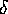is the declination andis the right ascension. The mass of each mass tracer is determined by an initial guess for the mass of M31, whereupon the celestial coordinates are adjusted to the centre of mass rest frame.

Also read from file are the initial values of the coefficients, i.e. the starting point for the search. As discussed earlier, this point is chosen as close as possible to where the solution is assumed to be, which, hopefully, will reduce the computing time. If one can assume that the system of mass tracers is cosmologically young; i.e. they are moving towards each other for the first time, the peculiar velocities are probably small. This indicates, by referring to equation (3.21), small values of the coefficients. Therefore, a preferable choice for initial values should be close to zero, both positive and negative. A common choice is actually. Details on the input files are given in Appendix A.4.

Usually the coefficients are given a common initial value, but it is sometimes practical to be able to specify a specific value for each coefficient. Hence, in the AVP code, one has the choice of either giving the same value to each coefficient, or reading individual values from file. This also gives us the freedom to halt and restart the computation at any point or to use the solution from one cosmological model as initial values for a search in another.

The next step is the initialization of some parameters and variables, e.g.from equation (3.36), and the trial functions (either 3.22 or 3.23). The latter ones are determined at the points in time, or rather points in the expansion parameter, determined by the integration method. As stated in Section 4.2, the integration method used here is the Gaussian Quadrature method, and the number of abscissas is consistently chosen to exceed the number of trial functions, N, by at least one. The abscissas and their respective weights are calculated as shown in equation (4.4), where the Gauss-Legendre abscissas and weights are determined by the gauleg routine given by Press90.

The iterative process can now commence. Any chosen optimizing method is the main part of this process, with underlying algorithms such as integration, linear searches, backtracking, and matrix inversion--depending on the choice of optimizing method. Two linear searches were tested: Davidon's method Walsh, which is a cubic interpolation method, and the secant method Shew, which is a version of the Newton-Raphson method in one dimension where the second derivative has been eliminated. There was practically no difference in the performance between these two methods, so the secant method was applied, mainly due to its simplicity. The source code for the secant method can be found in Appendix A.1

The linear searches include a number of calculations of both the action and its gradient at each iteration, which in some cases can take up a considerable amount of computing time. Therefore, the linear search is sometimes omitted, simply using a preassigned value for the step length factor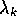(see equation 4.5), which either is gradually set to unity when closing in on the solution when applied for the Newton-like methods, or is just modified so that the action decreases at each iteration (Steepest Descent method). This may result in more iterations, but the total computing time can be reduced. The backtracking scheme is, in reality, just a more advanced version of these rather crude stepsize algorithms, where the step length is determined to be equal to or less than unity by fulfilling certain criteria for the decrease of the action (see equation 5.21). The computational effort of this method is less than for the linear search, but somewhat more than for the crude stepsize algorithms, and it only applies for the Newton-Raphson and the Secant method.

The Newton-Raphson optimizing method needs a matrix inversion algorithm; i.e. solving a linear system as shown in equation (4.18). There are several methods for this type of problem, e.g. Gauss-Jordan elimination, Gauss elimination with backsubstitution, LU decomposition, and Cholesky factorization. All these four methods have been tried here, and not surprisingly, since the matrix inverse are not needed per se, the LU decomposition and Cholesky factorization proved to be the most efficient. The Cholesky factorization is the better of the two, but has the limitation that the matrix must be positive definite. Therefore, the Cholesky factorization will only be used when the Hessian is positive definite, otherwise, the LU decomposition will be applied. More on these methods in Press.

The iterative process was terminated when the minimum point (or saddle point) is reached within a given accuracy or, optionally, when a maximum number of iterations is exceeded. A measure for the approach to a stationary point is when the norm of the gradient becomes sufficiently small, that is, when a pointis obtained withwhereis a small positive scalar. If the solution is to be determined to machine accuracy in double precision, the iterations are terminated when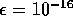, which means that the average error for each variable is about. This accuracy is somewhat extravagant, since the error in the input parameters and the error due to the truncation in the parameterization of the orbits in equation (3.21) is at least ten factor of ten larger. But, for some of the optimizing methods, e.g. the Newton-Raphson method, there is negligible extra computational cost in driving the solution to machine accuracy; when the accuracy of abouthas been reached, the method converge to machine accuracy in just a few iterations. For other optimizing methods with slower convergence, e.g. Steepest Descent, a more modest accuracy must be accepted in order to avoid impossibly long computing time.

After the termination of the iterative process, the code continues to calculate the orbits and physical velocities. In plotting the orbits, we are only interested in the comoving positions, which are given by (3.21); i.e.The trial functions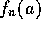can now be calculated at arbitrary times in the expansion parameter since there now is no need for any numerical integration. 500 equally spaced points in time were used here, which results in a very smooth curve.

As a test of the validity of the AVP orbits, a conventional N-body code is often used. Contrary to the AVP, these codes solve initial value problems, where both the velocity and position of each particle at a given point in time is needed. The N-body codes then calculate the orbits forward in time. Therefore, to be able to check the AVP orbits against a N-body program, we need both the position and velocity at a given time early in each mass tracer's history from the former as input to the latter, then in physical coordinates.(More on N-body codes in Section 5.2.) The physical positions are given by equation (2.5) and the physical velocities by equation (2.6).Thein the latter is either given by equation (3.33) or (3.34). The peculiar velocity given in the last term on the right hand side of equation (2.6) requires that the time derivatives of the comoving positions are determined. By equation (5.5), we havewhich enable us to determine the physical velocity at any given time. The physical velocities at the current time are of special interest, since they can be compared to observed radial velocities. For example, the observed value of the radial velocity of M31 can be used to determine its mass, and thereby determine the mass of all the other mass tracers in the system, since they are scaled to the mass of M31. This is one of the two procedures used to determine the mass of the system: the mass of M31 is adjusted until there is an acceptable fit between the observed and the predicted radial velocity of M31, which is -123 km sP-89. In the second procedure, one tries to get an best overall fit between observed and predicted radial velocities for several mass tracers by adjusting the mass of M31, using mass tracers that we have acceptably accurate observations of.

In this section, the orbits are intended to be compared with the solutions of P-89, which means that the coordinate system has to be rotated, so that the connecting line between the MW and M31 is aligned with the x-axis. Hence, both the positions and the velocities are adjusted to this transformed coordinate system. More on the presentation of the orbits in the next subsection.

After this slight adjustment, the output routine is being reached. Firstly, the number of iterations and the norm of the gradientsare written to screen. Secondly, the type of solution arrived at is being determined: minimum, maximum. or saddle point. This is done by looking at the eigenvalues of the Hessian matrix at the stationary point: if the eigenvalues are all positive, we have a minimum; if they are all positive, we have a maximum; and if there are both positive and negative eigenvalues, we are dealing with a saddle point. The eigenvalues are calculated by using the tred2 and tqli from Press90, where the former reduce the symmetric matrix to a tridiagonal system, while the latter determine the eigenvalues of such a tridiagonal matrix.

The closing stages of the AVP code has now been reached, were the results are written to file: the coefficients, the orbits (that is, the positions at 500 points in time), and the initial values used as input to the N-body code.

I close this section by recapitulating the expressions for the action and its derivative with respect to the coefficients: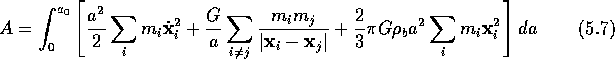and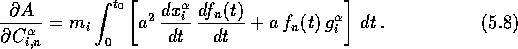where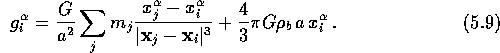Notice that this is the derivative prior to the integration by parts in equation (3.24). We also need an expression for the second derivative, used by the Newton-Raphson optimizing method. Derivation of (5.8) with respect to all the other coefficients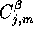gives: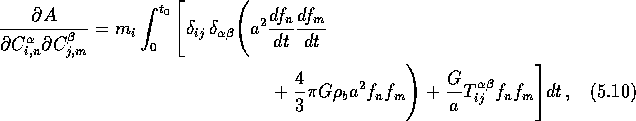wherewith, andbeing the common Kronecker delta. Note the difference between the subscript m and the mass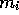.

In the equations above, the cutoff length c discussed in Section 3.1 is not included, but is being used in the calculations of the orbits. The purpose of the cutoff is to avoid singularities in the gravitational acceleration at zero separation, and to simulate spherical halos. The cutoff, often also referred to as a softening parameter, is introduced to the first term in equation (5.9) in the following way:The second term in equation (5.7) is being modified in the same manner. In this thesis the cutoff of P-90 is adapted; i.e.of the radiusgiven in equation (3.36). This is a relatively large cutoff, and should allow reasonably large halos.Next: Trial Orbits Up: Implementation of the AVP Previous: Implementation of the AVP

Trond Hjorteland
Mon Jul 5 02:59:28 MET DST 1999